## A small computational thought experiment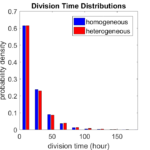In Macklin (2017), I briefly touched on a simple computational thought experiment that shows that for a group of homogeneous cells, you can observe substantial heterogeneity in cell behavior. This “thought experiment” is part of a broader preview and discussion of a fantastic paper by Linus Schumacher, Ruth Baker, and Philip Maini published in Cell Systems, where they showed that a migrating collective homogeneous cells can show heterogeneous behavior when quantitated with new migration metrics. I highly encourage you to check out their work!

In this blog post, we work through my simple thought experiment in a little more detail.

Note: If you want to reference this blog post, please cite the Cell Systems preview article:

P. Macklin, When seeing isn’t believing: How math can guide our interpretation of measurements and experiments. Cell Sys., 2017 (in press). DOI: 10.1016/j.cells.2017.08.005

### The thought experiment

Consider a simple (and widespread) model of a population of cycling cells: each virtual cell (with index i) has a single “oncogene” $$r_i$$ that sets the rate of progression through the cycle. Between now (t) and a small time from now ( $$t+\Delta t$$), the virtual cell has a probability $$r_i \Delta t$$ of dividing into two daughter cells. At the population scale, the overall population growth model that emerges from this simple single-cell model is:
$\frac{dN}{dt} = \langle r\rangle N,$
where $$\langle r \rangle$$ the mean division rate over the cell population, and is the number of cells. See the discussion in the supplementary information for Macklin et al. (2012).

Now, suppose (as our thought experiment) that we could track individual cells in the population and track how long it takes them to divide. (We’ll call this the division time.) What would the distribution of cell division times look like, and how would it vary with the distribution of the single-cell rates $$r_i$$?

### Mathematical method

In the Matlab script below, we implement this cell cycle model as just about every discrete model does. Here’s the pseudocode:

t = 0;
while( t < t_max )
for i=1:Cells.size()
u = random_number();
if( u < Cells[i].birth_rate * dt )
Cells[i].division_time = Cells[i].age;
Cells[i].divide();
end
end
t = t+dt;
end


That is, until we’ve reached the final simulation time, loop through all the cells and decide if they should divide: For each cell, choose a random number between 0 and 1, and if it’s smaller than the cell’s division probability ($$r_i \Delta t$$), then divide the cell and write down the division time.

As an important note, we have to track the same cells until they all divide, rather than merely record which cells have divided up to the end of the simulation. Otherwise, we end up with an observational bias that throws off our recording. See more below.

### The sample code

http://MathCancer.org/files/matlab/thought_experiment_matlab(Macklin_Cell_Systems_2017).zip

Extract all the files, and run “thought_experiment” in Matlab (or Octave, if you don’t have a Matlab license or prefer an open source platform) for the main result.

All these Matlab files are available as open source, under the GPL license (version 3 or later).

### Results and discussion

First, let’s see what happens if all the cells are identical, with $$r = 0.05 \textrm{ hr}^{-1}$$. We run the script, and track the time for each of 10,000 cells to divide. As expected by theory (Macklin et al., 2012) (but perhaps still a surprise if you haven’t looked), we get an exponential distribution of division times, with mean time $$1/\langle r \rangle$$: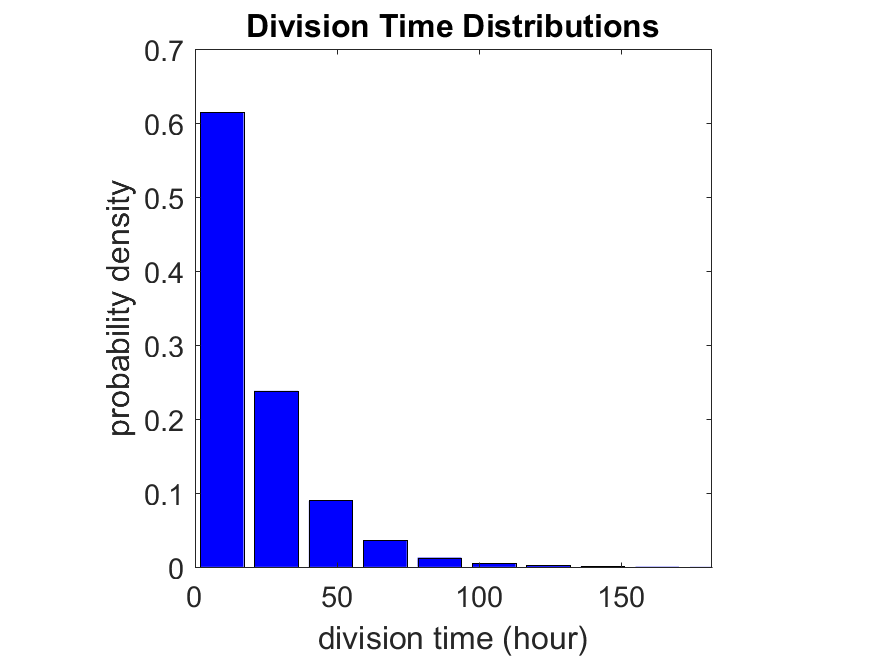So even in this simple model, a homogeneous population of cells can show heterogeneity in their behavior. Here’s the interesting thing: let’s now give each cell its own division parameter $$r_i$$ from a normal distribution with mean $$0.05 \textrm{ hr}^{-1}$$ and a relative standard deviation of 25%: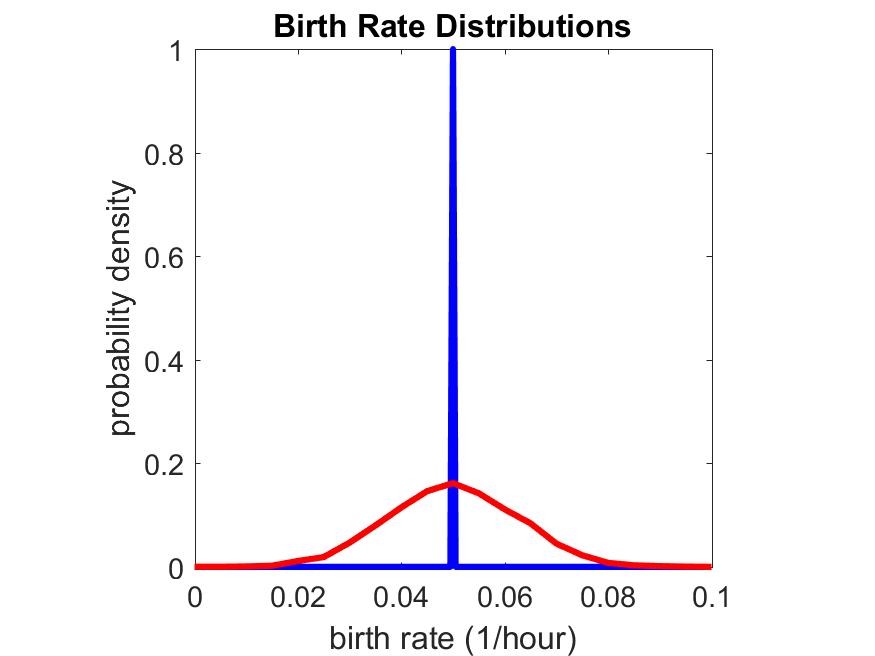If we repeat the experiment, we get the same distribution of cell division times!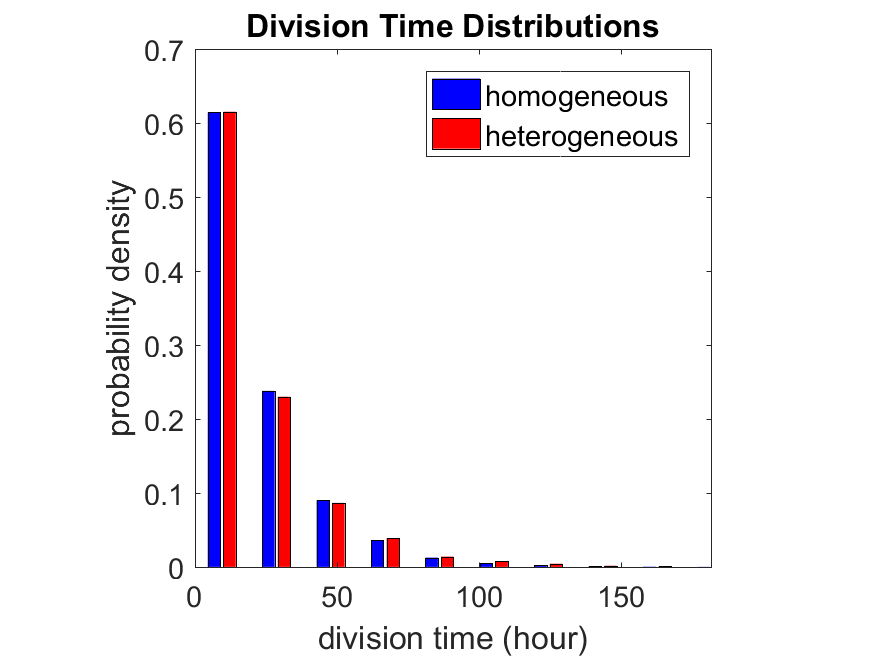So in this case, based solely on observations of the phenotypic heterogeneity (the division times), it is impossible to distinguish a “genetically” homogeneous cell population (one with identical parameters) from a truly heterogeneous population. We would require other metrics, like tracking changes in the mean division time as cells with a higher $$r_i$$ out-compete the cells with lower $$r_i$$.

Lastly, I want to point out that caution is required when designing these metrics and single-cell tracking. If instead we had tracked all cells throughout the simulated experiment, including new daughter cells, and then recorded the first 10,000 cell division events, we would get a very different distribution of cell division times: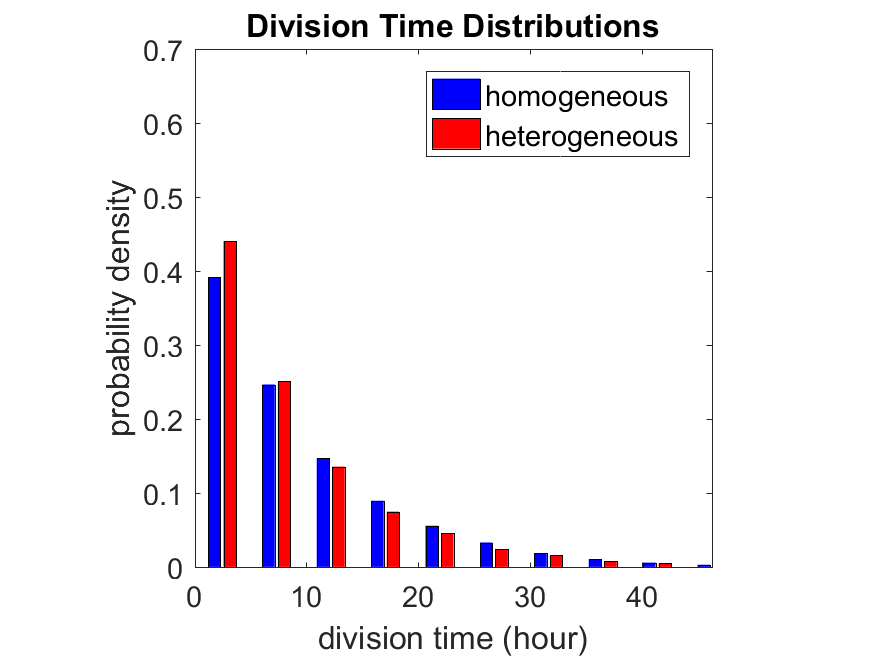By only recording the division times for the cells that have divided, and not those that haven’t, we bias our observations towards cells with shorter division times. Indeed, the mean division time for this simulated experiment is far lower than we would expect by theory. You can try this one by running “bad_thought_experiment”.

This post is an expansion of our recent preview in Cell Systems in Macklin (2017):

P. Macklin, When seeing isn’t believing: How math can guide our interpretation of measurements and experiments. Cell Sys., 2017 (in press). DOI: 10.1016/j.cells.2017.08.005

And the original work on apparent heterogeneity in collective cell migration is by Schumacher et al. (2017):

L. Schumacher et al., Semblance of Heterogeneity in Collective Cell MigrationCell Sys., 2017 (in press). DOI: 10.1016/j.cels.2017.06.006

You can read some more on relating exponential distributions and Poisson processes to common discrete mathematical models of cell populations in Macklin et al. (2012):

P. Macklin, et al., Patient-calibrated agent-based modelling of ductal carcinoma in situ (DCIS): From microscopic measurements to macroscopic predictions of clinical progressionJ. Theor. Biol. 301:122-40, 2012. DOI: 10.1016/j.jtbi.2012.02.002.

Lastly, I’d be delighted if you took a look at the open source software we have been developing for 3-D simulations of multicellular systems biology:

http://OpenSource.MathCancer.org

And you can always keep up-to-date by following us on Twitter: @MathCancer.

## MathCancer C++ Style and Practices Guide

As PhysiCell, BioFVM, and other open source projects start to gain new users and contributors, it’s time to lay out a coding style. We have three goals here:

1. Consistency: It’s easier to understand and contribute to the code if it’s written in a consistent way.
2. Readability: We want the code to be as readable as possible.
3. Reducing errors: We want to avoid coding styles that are more prone to errors. (e.g., code that can be broken by introducing whitespace).

So, here is the guide (revised June 2017). I expect to revise this guide from time to time.

### Place braces on separate lines in functions and classes.

I find it much easier to read a class if the braces are on separate lines, with good use of whitespace. Remember: whitespace costs almost nothing, but reading and understanding (and time!) are expensive.

#### DON’T

class Cell{
public:
double some_variable;
bool some_extra_variable;

Cell(); };

class Phenotype{
public:
double some_variable;
bool some_extra_variable;

Phenotype();
};


#### DO:

class Cell
{
public:
double some_variable;
bool some_extra_variable;

Cell();
};

class Phenotype
{
public:
double some_variable;
bool some_extra_variable;

Phenotype();
};


### Enclose all logic in braces, even when optional.

In C/C++, you can omit the curly braces in some cases. For example, this is legal

if( distance > 1.5*cell_radius )
interaction = false;
force = 0.0; // is this part of the logic, or a separate statement?
error = false;


However, this code is ambiguous to interpret. Moreover, small changes to whitespace–or small additions to the logic–could mess things up here. Use braces to make the logic crystal clear:

#### DON’T

if( distance > 1.5*cell_radius )
interaction = false;
force = 0.0; // is this part of the logic, or a separate statement?
error = false;

if( condition1 == true )
do_something1 = true;
elseif( condition2 == true )
do_something2 = true;
else
do_something3 = true;


#### DO

if( distance > 1.5*cell_radius )
{
interaction = false;
force = 0.0;
}
error = false;

if( condition1 == true )
{ do_something1 = true; }
elseif( condition2 == true )
{ do_something2 = true; }
else
{ do_something3 = true; }


### Put braces on separate lines in logic, except for single-line logic.

This style rule relates to the previous point, to improve readability.

#### DON’T

if( distance > 1.5*cell_radius ){
interaction = false;
force = 0.0; }

if( condition1 == true ){ do_something1 = true; }
elseif( condition2 == true ){
do_something2 = true; }
else
{ do_something3 = true; error = true; }


#### DO

if( distance > 1.5*cell_radius )
{
interaction = false;
force = 0.0;
}

if( condition1 == true )
{ do_something1 = true; } // this is fine
elseif( condition2 == true )
{
do_something2 = true; // this is better
}
else
{
do_something3 = true;
error = true;
}


See how much easier that code is to read? The logical structure is crystal clear, and adding more to the logic is simple.

### End all functions with a return, even if void.

For clarity, definitively state that a function is done by using return.

#### DON’T

void my_function( Cell& cell )
{
cell.phenotype.volume.total *= 2.0;
cell.phenotype.death.rates = 0.02;
// Are we done, or did we forget something?
// is somebody still working here?
}


#### DO

void my_function( Cell& cell )
{
cell.phenotype.volume.total *= 2.0;
cell.phenotype.death.rates = 0.02;
return;
}


### Use tabs to indent the contents of a class or function.

This is to make the code easier to read. (Unfortunately PHP/HTML makes me use five spaces here instead of tabs.)

#### DON’T

class Secretion
{
public:
std::vector<double> secretion_rates;
std::vector<double> uptake_rates;
std::vector<double> saturation_densities;
};

void my_function( Cell& cell )
{
cell.phenotype.volume.total *= 2.0;
cell.phenotype.death.rates = 0.02;
return;
}


#### DO

class Secretion
{
public:
std::vector<double> secretion_rates;
std::vector<double> uptake_rates;
std::vector<double> saturation_densities;
};

void my_function( Cell& cell )
{
cell.phenotype.volume.total *= 2.0;
cell.phenotype.death.rates = 0.02;
return;
}


### Use a single space to indent public and other keywords in a class.

This gets us some nice formatting in classes, without needing two tabs everywhere.

#### DON’T

class Secretion
{
public:
std::vector<double> secretion_rates;
std::vector<double> uptake_rates;
std::vector<double> saturation_densities;
}; // not enough whitespace

class Errors
{
private:
public:
std::string error_message;
int error_code;
}; // too much whitespace!


#### DO

class Secretion
{
private:
public:
std::vector<double> secretion_rates;
std::vector<double> uptake_rates;
std::vector<double> saturation_densities;
};

class Errors
{
private:
public:
std::string error_message;
int error_code;
};


### Avoid arcane operators, when clear logic statements will do.

It can be difficult to decipher code with statements like this:

phenotype.volume.fluid=phenotype.volume.fluid<0?0:phenotype.volume.fluid;


Moreover, C and C++ can treat precedence of ternary operators very differently, so subtle bugs can creep in when using the “fancier” compact operators. Variations in how these operators work across languages are an additional source of error for programmers switching between languages in their daily scientific workflows. Wherever possible (and unless there is a significant performance reason to do so), use clear logical structures that are easy to read even if you only dabble in C/C++. Compiler-time optimizations will most likely eliminate any performance gains from these goofy operators.

#### DON’T

// if the fluid volume is negative, set it to zero
phenotype.volume.fluid=phenotype.volume.fluid<0.0?0.0:pCell->phenotype.volume.fluid;


#### DO

if( phenotype.volume.fluid < 0.0 )
{
phenotype.volume.fluid = 0.0;
}


Here’s the funny thing: the second logic is much clearer, and it took fewer characters, even with extra whitespace for readability!

### Pass by reference where possible.

Passing by reference is a great way to boost performance: we can avoid (1) allocating new temporary memory, (2) copying data into the temporary memory, (3) passing the temporary data to the function, and (4) deallocating the temporary memory once finished.

#### DON’T

double some_function( Cell cell )
{
return = cell.phenotype.volume.total + 3.0;
}
// This copies cell and all its member data!


#### DO

double some_function( Cell& cell )
{
return = cell.phenotype.volume.total + 3.0;
}
// This just accesses the original cell data without recopying it.


### Where possible, pass by reference instead of by pointer.

There is no performance advantage to passing by pointers over passing by reference, but the code is simpler / clearer when you can pass by reference. It makes code easier to write and understand if you can do so. (If nothing else, you save yourself character of typing each time you can replace “->” by “.”!)

#### DON’T

double some_function( Cell* pCell )
{
return = pCell->phenotype.volume.total + 3.0;
}
// Writing and debugging this code can be error-prone.


#### DO

double some_function( Cell& cell )
{
return = cell.phenotype.volume.total + 3.0;
}
// This is much easier to write.


### Be careful with static variables. Be thread safe!

PhysiCell relies heavily on parallelization by OpenMP, and so you should write functions under the assumption that they may be executed many times simultaneously. One easy source of errors is in static variables:

#### DON’T

double some_function( Cell& cell )
{
static double four_pi = 12.566370614359172;
static double output;
output *= output;
output *= four_pi;
return output;
}
// If two instances of some_function are running, they will both modify
// the *same copy* of output


#### DO

double some_function( Cell& cell )
{
static double four_pi = 12.566370614359172;
double output;
output *= output;
output *= four_pi;
return output;
}
// If two instances of some_function are running, they will both modify
// the their own copy of output, but still use the more efficient, once-
// allocated copy of four_pi. This one is safe for OpenMP.


### Use std:: instead of “using namespace std”

PhysiCell uses the BioFVM and PhysiCell namespaces to avoid potential collision with other codes. Other codes using PhysiCell may use functions that collide with the standard namespace. So, we formally use std:: whenever using functions in the standard namespace.

#### DON’T

using namespace std;

cout << "Hi, Mom, I learned to code today!" << endl;
string my_string = "Cheetos are good, but Doritos are better.";
cout << my_string << endl;

vector<double> my_vector;
vector.resize( 3, 0.0 );


#### DO

std::cout << "Hi, Mom, I learned to code today!" << std::endl;
std::string my_string = "Cheetos are good, but Doritos are better.";
std::cout << my_string << std::endl;

std::vector<double> my_vector;
my_vector.resize( 3, 0.0 );


### Camelcase is ugly. Use underscores.

This is purely an aesthetic distinction, but CamelCaseCodeIsUglyAndDoYouUseDNAorDna?

#### DON’T

double MyVariable1;
bool ProteinsInExosomes;
int RNAtranscriptionCount;

void MyFunctionDoesSomething( Cell& ImmuneCell );


#### DO

double my_variable1;
bool proteins_in_exosomes;
int RNA_transcription_count;

void my_function_does_something( Cell& immune_cell );


### Use capital letters to declare a class. Use lowercase for instances.

To help in readability and consistency, declare classes with capital letters (but no camelcase), and use lowercase for instances of those classes.

#### DON’T

class phenotype;

class cell
{
public:
std::vector<double> position;
phenotype Phenotype;
};

class ImmuneCell : public cell
{
public:
std::vector<double> surface_receptors;
};

void do_something( cell& MyCell , ImmuneCell& immuneCell );

cell Cell;
ImmuneCell MyImmune_cell;

do_something( Cell, MyImmune_cell );


#### DO

class Phenotype;

class Cell
{
public:
std::vector<double> position;
Phenotype phenotype;
};

class Immune_Cell : public Cell
{
public:
std::vector<double> surface_receptors;
};

void do_something( Cell& my_cell , Immune_Cell& immune_cell );

Cell cell;
Immune_Cell my_immune_cell;

do_something( cell, my_immune_cell );


## DCIS modeling paper accepted

Recently, I wrote about a major work we submitted to the Journal of Theoretical Biology: “Patient-calibrated agent-based modelling of ductal carcinoma in situ (DCIS): From microscopic measurements to macroscopic predictions of clinical progression.”

I am pleased to report that our paper has now been accepted.  You can download the accepted preprint here. We also have a lot of supplementary material, including simulation movies, simulation datasets (for 0, 15, 30, adn 45 days of growth), and open source C++ code for postprocessing and visualization.

I discussed the results in detail here, but here’s the short version:

1. We use a mechanistic, agent-based model of individual cancer cells growing in a duct. Cells are moved by adhesive and repulsive forces exchanged with other cells and the basement membrane.  Cell phenotype is controlled by stochastic processes.
2. We constrained all parameter expected to be relatively independent of patients by a careful analysis of the experimental biological and clinical literature.
3. We developed the very first patient-specific calibration method, using clinically-accessible pathology.  This is a key point in future patient-tailored predictions and surgical/therapeutic planning.
4. The model made numerous quantitative predictions, such as:
1. The tumor grows at a constant rate, between 7 to 10 mm/year. This is right in the middle of the range reported in the clinic.
2. The tumor’s size in mammgraphy is linearly correlated with the post-surgical pathology size.  When we linearly extrapolate our correlation across two orders of magnitude, it goes right through the middle of a cluster of 87 clinical data points.
3. The tumor necrotic core has an age structuring: with oldest, calcified material in the center, and newest, most intact necrotic cells at the outer edge.
4. The appearance of a “typical” DCIS duct cross-section varies with distance from the leading edge; all types of cross-sections predicted by our model are observed in patient pathology.
5. The model also gave new insight on the underlying biology of breast cancer, such as:
1. The split between the viable rim and necrotic core (observed almost universally in pathology) is not just an artifact, but an actual biomechanical effect from fast necrotic cell lysis.
2. The constant rate of tumor growth arises from the biomechanical stress relief provided by lysing necrotic cells. This points to the critical role of intracellular and intra-tumoral water transport in determining the qualitative and quantitative behavior of tumors.
3. Pyknosis (nuclear degradation in necrotic cells), must occur at a time scale between that of cell lysis (on the order of hours) and cell calcification (on the order of weeks).
4. The current model cannot explain the full spectrum of calcification types; other biophysics, such as degradation over a long, 1-2 month time scale, must be at play.
I hope you enjoy this article and find it useful. It is our hope that it will help drive our field from qualitative theory towards quantitative, patient-tailored predictions.
Direct link to the preprint: http://www.mathcancer.org/Publications.php#macklin12_jtb
I want to express my greatest thanks to my co-authors, colleagues, and the editorial staff at the Journal of Theoretical Biology.

## Now hiring: Postdoctoral Researcher

I just posted a job opportunity for a postdoctoral researcher for computational modeling of breast, prostate, and metastatic cancer, with a heavy emphasis on calibrating (and validating!) to in vitro, in vivo, and clinical data.

If you’re a talented computational modeler and have a passion for applying mathematics to make a difference in clinical care, please read the job posting and apply!

(Note: Interested students in the Los Angeles/Orange County area may want to attend my applied math seminar talk at UCI next week to learn more about this work.)